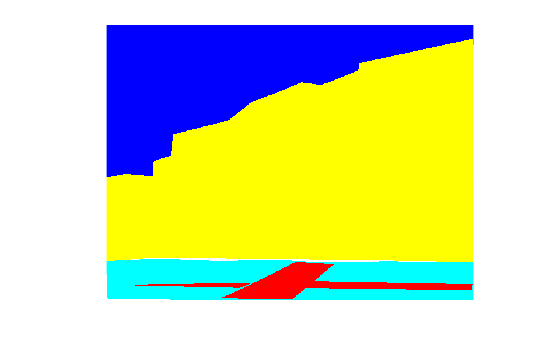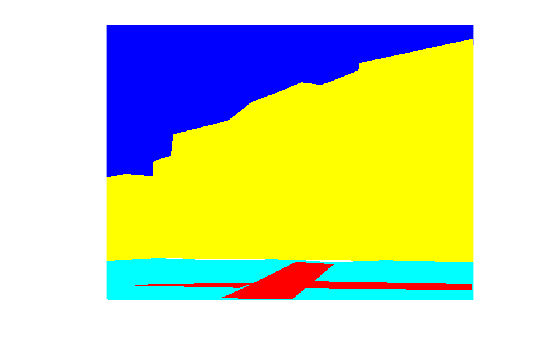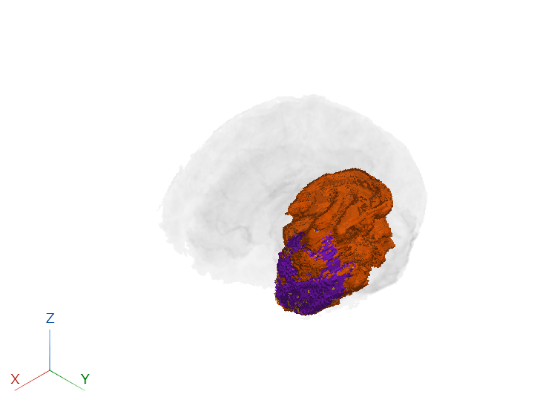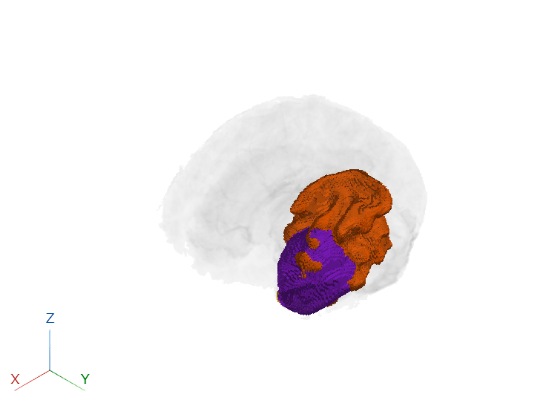Main Content

# modefilt

2-D and 3-D mode filtering

## Syntax

``B = modefilt(A)``
``B = modefilt(A,filtsize)``
``B = modefilt(___,padopt)``

## Description

example

````B = modefilt(A)` performs mode filtering on the 2-D image or 3-D volume `A`. Each output pixel in `B` contains the mode (most frequently occurring value) in the neighborhood around the corresponding pixel in `A`. If `A` is 2-D, `modefilt` uses a 3-by-3 mode filter. If `A` is 3-D, `modefilt` uses a 3-by-3-by-3 mode filter. `modefilt` pads `A` by mirroring border elements.Mode filtering can be useful for processing categorical data, where other types of filtering, such as median filtering, are not available.```
````B = modefilt(A,filtsize)` also specifies the size of the filter neighborhood. `filtsize` is a vector of positive, odd integers. When `A` is 2-D, specify `filtsize` as a 1-by-2 vector. When `A` is 3-D, specify `filtsize` as a 1-by-3 vector. ```
````B = modefilt(___,padopt)` also specifies how `modefilt` pads array boundaries. ```

## Examples

collapse all

Load an image (`img`) and the corresponding categorical labeled version of the image (`label`) into the workspace.

`load buildingPixelLabeled;`

View the original image, `img`.

`imshow(img)`View the categorical labeled image, `label`. The categorical image labels four separate categories: sky, grass, building, and sidewalk. For viewing, convert these categories to colors using the `label2rgb` function.

`imshow(label2rgb(label))`Perform mode filtering on the categorical labeled image, `label`, using the default filter size and padding method.

` b = modefilt(label);`

View the filtered categorical labeled image, `b`. In the filtered image, the edges between labeled regions are more distinct.

``` figure imshow(label2rgb(b));```Read a labeled volume of an MRI. The volume is stored in the workspace variable `label`.

```load(fullfile(toolboxdir('images'),'imdata','BrainMRILabeled', ... 'labels','label_001.mat'));```

Display the labeled volume. For clarity, add a title to the display.

```ViewPnl = uipanel(figure,'Title','Labeled Volume'); labelvolshow(label,'Parent',ViewPnl);```Perform mode filtering on the labeled volume, specifying the size of the filter.

`labelOut = modefilt(label,[5 5 5]);`

Display the filtered labeled volume. For clarity, add a title to the display.

```ViewPnlFiltered = uipanel(figure,'Title','Mode Filtered Labeled Volume'); labelvolshow(labelOut,'Parent',ViewPnlFiltered);```## Input Arguments

collapse all

2-D image or 3-D volume, specified as a categorical, logical, or numeric array.

Data Types: `single` | `double` | `int8` | `int16` | `int32` | `uint8` | `uint16` | `uint32` | `logical` | `categorical`

Size of filter, specified as a vector of positive odd integers. For 2-D images, specify a vector of the form `[height width]`. The default for 2-D images is `[3 3]`. For 3-D volumes, specify a vector of the form `[height width depth]`. The default for 3-D volumes is ```[3 3 3]```.

Data Types: `single` | `double` | `int8` | `int16` | `int32` | `int64` | `uint8` | `uint16` | `uint32` | `uint64`

Padding method, specified as one of the following values.

ValueDescription
`'symmetric'`Pad array with a mirror reflection of itself.
`'replicate'`Pad array by repeating border elements.
`'zeros'`Pad array with `0`s for numeric data or with `<undefined>`s for categorical data.

Example: `labelOut = modefilt(label,'replicate');`

Data Types: `char` | `string`

## Output Arguments

collapse all

Filtered image or volume, returned as a numeric array of the same size and class as the input image `A`.

## Tips

• When the neighborhood has more than one pixel in a tie for the mode value, the function uses the following tie-breaking algorithm:

• If the center pixel is one of the mode values in the tie, the function uses this value.

• If the center pixel is not one of the mode values in the tie, the function uses the mode with the smallest numeric value.

• For categorical input, the function chooses the first category (among the categories tied for mode) that appears in the list returned by `categories(A)`.

• `modefilt` treats RGB images as 3-D volumes. To do channel-wise filtering of an RGB image, specify `filtsize` as ```[3 3 1]```, as in this code: `b = modefilt(a,[3 3 1]); `.

## See Also

Introduced in R2020a

Download ebook